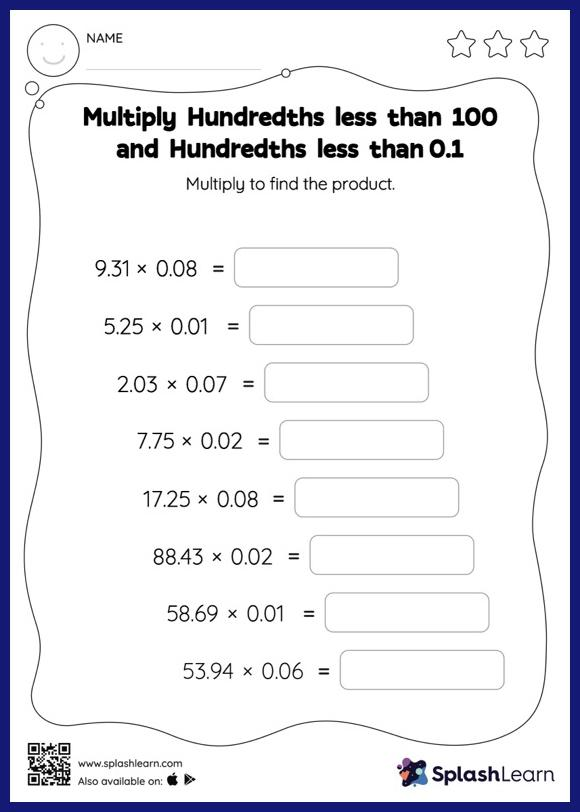# Multiply Hundredths less than 100 and Hundredths less than 0.1: Horizontal Multiplication Worksheet

Home > Multiply Hundredths less than 100 and Hundredths less than 0.1: Horizontal MultiplicationMultiply hundredths less than 100 and hundredths less than 0.1 Worksheet helps students develop proficiency with multiplication. When students multiply two decimals, they first multiply as if there is no decimal. Then they count the number of decimal digits in each number and put the same number of digits after the decimal point in the product. Students practice this concept in great detail with this multiply hundredths less than 100 and hundredths less than 0.1 Worksheet. In this worksheet, students practice solving problems written in the horizontal format. How numbers are laid out in a problem affects the method a student employs to solve it. Therefore to develop actual fluency and mastery of multiple strategies, students must practice different formats.